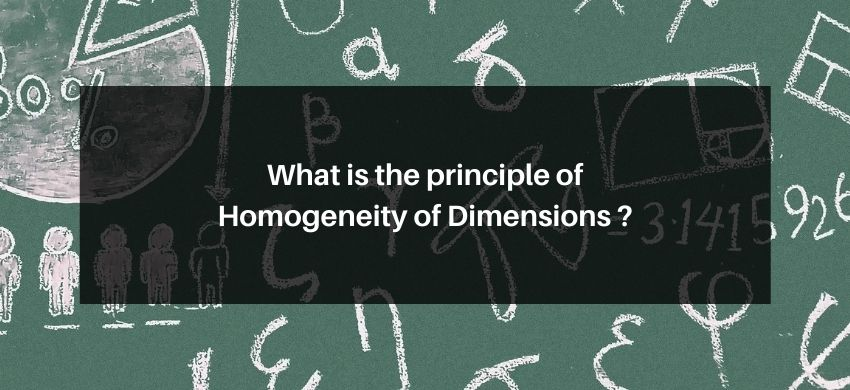Most Affordable JEE | NEET | 8,9,10 Preparation by Kota's Top IITian Doctor Faculties

# What is the principle of Homogeneity of Dimensions ?## Principle of Homogeneity of Dimensions

The dimension of physical quantity on the left-hand side of the dimensional equation should equal to the net dimensions of all physical quantities on the right-hand side of it.

Ex. If in the form $x=3 y z^{2}, x$ and $z$ represent electrical capacitances and magnetic induction the calculate the dimensional equation of $\mathrm{y}$.

Sol. By the principle of homogeneity of dimension

Dimension equation of $x \quad=$ Dimension equation of $\left(3 y z^{2}\right)$

$\mathrm{M}^{-1} \mathrm{~L}^{-2} \mathrm{~T}^{4} \mathrm{~A}^{2} \quad=$ Dimension equation of $(\mathrm{y}) \times\left(\mathrm{M}^{1} \mathrm{~L}^{0} \mathrm{~T}^{-2} \mathrm{~A}^{-1}\right)^{2}$

Dimension of $(y) \quad=M^{-3} L^{-2} T^{8} A^{4}$

Ex. In the formula; $N=-D\left[\frac{n_{2}-n_{1}}{x_{2}-x_{1}}\right], D=$ diffusion coefficient, $n_{1}$ and $n_{2}$ is number of molecules in unit volume along $x_{1}$ and $x_{2}$. Which represents distances where $N$ is number of molecules passing through per unit area per unit time Calculate dimensional equation of D.

Sol. By Homogeneity theory of Dimension

Dimension of $(N)=$ Dimension of $D \times \frac{\text { Dimension of }\left(n_{2}-n_{1}\right)}{\text { Dimension of }\left(x_{2}-x_{1}\right)}$

$\frac{1}{\mathrm{~L}^{2} \mathrm{~T}}=$ Dimension of $\mathrm{D} \times \frac{\mathrm{L}^{-3}}{\mathrm{~L}}$

$\Rightarrow \quad$ Dimension of ' $D$ ' $\quad=\frac{L}{L^{-3} \times L^{2} T}=\frac{L^{2}}{T}=L^{2} T^{-1}$